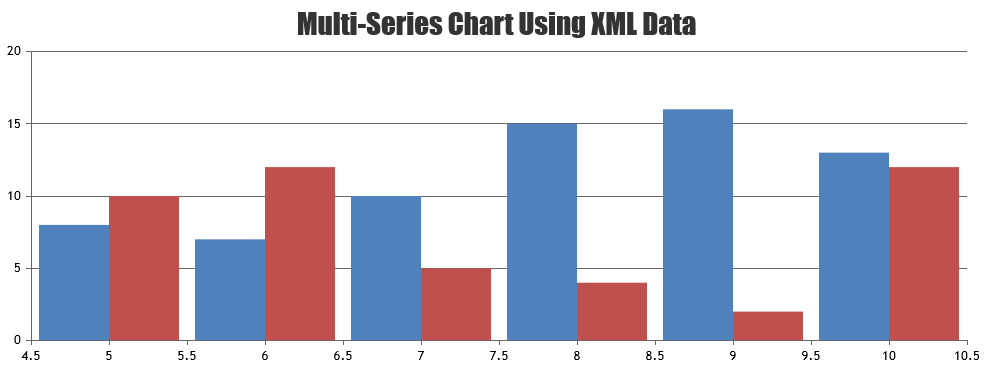Home Forums Chart Support multiple sets of datapoints

# multiple sets of datapoints

Viewing 2 posts - 1 through 2 (of 2 total)
• #36810

I was looking at this example:
https://canvasjs.com/docs/charts/how-to/create-charts-xml-data-ajax/

Is there a way to create multiple sets of datapoints from an XML file?

#36839

It is possible to create a multi-series chart with data from an XML file by looping through the XML data as shown in the code-snippet below,

``````\$(data).find("point").each(function () {
var \$dataPoint = \$(this);
var x = \$dataPoint.find("x").text();
var y1 = \$dataPoint.find("y1").text();
var y2 = \$dataPoint.find("y2").text();
dps1.push({x: parseFloat(x), y: parseFloat(y1)});
dps2.push({x: parseFloat(x), y: parseFloat(y2)});
});``````

The format of the dataPoints in the XML file (e.g. data.xml) should be:

``````<point>
<x>5</x>
<y1>8</y1>
<y2>10</y2>
</point>``````

Kindly take a look at this CodeSandbox for a working example.